# ARIMA模型预测CO2浓度时间序列-python实现

Python中可用的一种用于建模和预测时间序列的未来点的方法称为 SARIMAX，它表示带有季节性回归的 季节性自回归综合移动平均线。在这里，我们将主要关注ARIMA，用于拟合时间序列数据以更好地理解和预测时间序列中的未来点。

# 第1步-安装软件包

``````cd environments

. my_env/bin/activate``````

``````mkdir ARIMA
cd ARIMA ``````

``pip install pandas numpy statsmodels matplotlib ``

# 第2步-导入包并加载数据

``````mkdir ARIMA
cd ARIMA ``````

``pip install pandas numpy statsmodels matplotlib ``

# 第2步-导入包并加载数据

``y = data.data``

``````# “ MS”字符串按月初将数据分组到存储中
y = y['co2'].resample('MS').mean()

# 填充缺失值
y = y.fillna(y.bfill()) ``````
``````Output
co2
1958-03-01  316.100000
1958-04-01  317.200000
1958-05-01  317.433333
...
2001-11-01  369.375000
2001-12-01  371.020000``````

``plt.show()``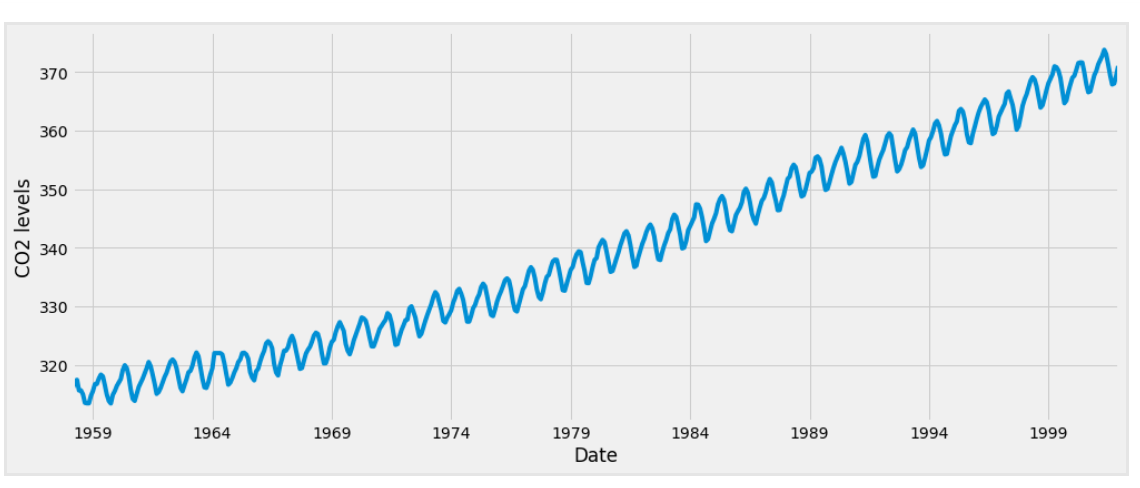# 第3步-ARIMA时间序列模型

• `p` 是模型的 自回归 部分。它使我们能够将过去值的影响纳入模型。从直觉上讲，这类似于声明如果过去三天天气一直温暖，明天可能会温暖。
• `d` 是 模型的差分部分。包含了要应用于时间序列的差分量（即，要从当前值中减去的过去时间点的数量）。从直觉上讲，这类似于如果最近三天的温差很小，则明天的温度可能相同。
• `q` 是 模型的 _移动平均_部分。这使我们可以将模型的误差设置为过去在先前时间点观察到的误差值的线性组合。

# 第4步-ARIMA时间序列模型的参数选择

``````#定义p，d和q参数，使其取0到2之间的任何值
p = d = q = range(0, 2)

# 生成p、q和q三元组的所有不同组合
pdq = list(itertools.product(p, d, q))

# 生成所有不同的季节性p，q和q组合
seasonal_pdq = [(x, x, x, 12) for x in list(itertools.product(p, d, q))] ``````
``````Output
Examples of parameter combinations for Seasonal ARIMA...
SARIMAX: (0, 0, 1) x (0, 0, 1, 12)
SARIMAX: (0, 0, 1) x (0, 1, 0, 12)
SARIMAX: (0, 1, 0) x (0, 1, 1, 12)
SARIMAX: (0, 1, 0) x (1, 0, 0, 12)``````

# 下面的代码块通过参数组合进行迭代，并使用中的 `SARIMAX` 函数 `statsmodels` 来拟合相应的Season ARIMA模型。拟合每个 `SARIMAX()`模型后，代码将输出出它们各自的 `AIC` 分数。

``````warnings.filterwarnings("ignore") # 指定忽略警告消息

try:
mod = sm.tsa.statespace.SARIMAX(y,
order=param,
seasonal_order=param_seasonal,
enforce_stationarity=False,
enforce_invertibility=False) ``````

``````Output
SARIMAX(0, 0, 0)x(0, 0, 1, 12) - AIC:6787.3436240402125
SARIMAX(0, 0, 0)x(0, 1, 1, 12) - AIC:1596.711172764114
SARIMAX(0, 0, 0)x(1, 0, 0, 12) - AIC:1058.9388921320026
SARIMAX(0, 0, 0)x(1, 0, 1, 12) - AIC:1056.2878315690562
SARIMAX(0, 0, 0)x(1, 1, 0, 12) - AIC:1361.6578978064144
SARIMAX(0, 0, 0)x(1, 1, 1, 12) - AIC:1044.7647912940095
...
...
...
SARIMAX(1, 1, 1)x(1, 0, 0, 12) - AIC:576.8647112294245
SARIMAX(1, 1, 1)x(1, 0, 1, 12) - AIC:327.9049123596742
SARIMAX(1, 1, 1)x(1, 1, 0, 12) - AIC:444.12436865161305
SARIMAX(1, 1, 1)x(1, 1, 1, 12) - AIC:277.7801413828764``````

# 步骤5 —拟合ARIMA时间序列模型

`` results = mod.fit() ``
``````Output
==============================================================================
coef    std err          z      P>|z|      [0.025      0.975]
------------------------------------------------------------------------------
ar.L1          0.3182      0.092      3.443      0.001       0.137       0.499
ma.L1         -0.6255      0.077     -8.165      0.000      -0.776      -0.475
ar.S.L12       0.0010      0.001      1.732      0.083      -0.000       0.002
ma.S.L12      -0.8769      0.026    -33.811      0.000      -0.928      -0.826
sigma2         0.0972      0.004     22.634      0.000       0.089       0.106
==============================================================================``````

`summary` 输出结果产生的 属性 `SARIMAX` 返回了大量信息，但是我们将注意力集中在系数上。 `coef` 列显示每个函数的权重（即重要性）以及每个函数如何影响时间序列。 `P>|z|` 列告知我们每个特征权重的重要性。在这里，每个权重的p值都小于或接近 `0.05`，因此将所有权重保留在我们的模型中是合理的。

``plt.show()``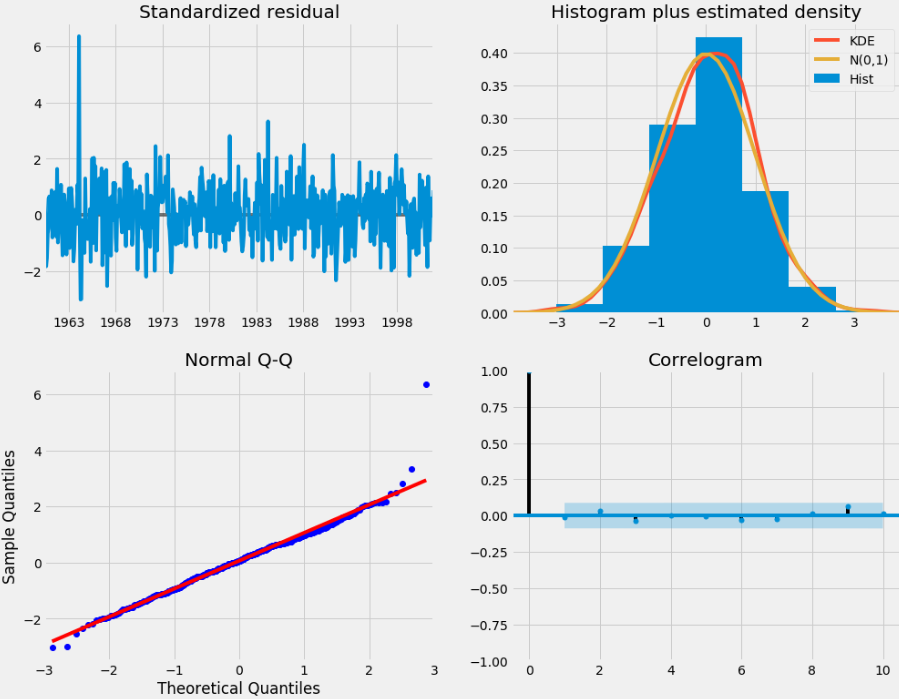• 在右上角的图中，我们看到红线 `KDE` 靠近 `N(0,1)` 红线，（其中 `N(0,1)`）是均值`0` 和标准偏差 为的正态分布。这很好地表明了残差呈正态分布。
• 左下方的 qq图显示，残差（蓝色点）分布遵循从标准正态分布。同样，表明残差是正态分布的。
• 随时间推移的残差（左上图）没有显示任何明显的季节性变化，而是白噪声。右下方的自相关（即相关图）图证实了这一点，该图表明时间序列残差有较低的相关性。

# 第6步-验证预测

``pred_ci = pred.conf_int()``

`````` ax.fill_between(pred_ci.index,
pred_ci.iloc[:, 0],
pred_ci.iloc[:, 1], color='k', alpha=.2)

plt.show()``````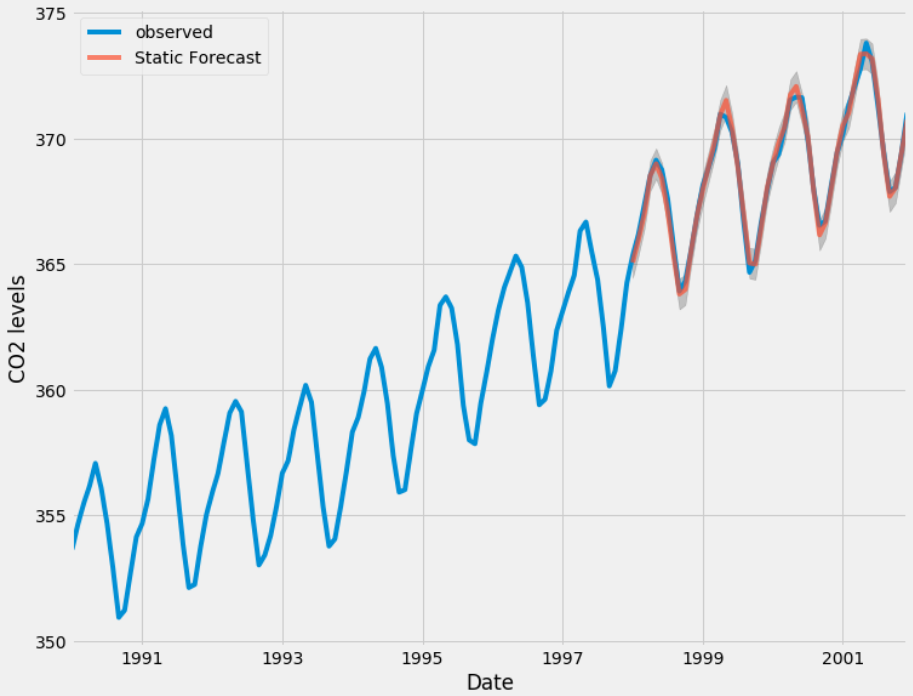``````y_truth = y['1998-01-01':]

# 计算均方误差
mse = ((y_forecasted - y_truth) ** 2).mean() ``````
``````Output

``````# 提取时间序列的预测值和真实值
y_forecasted = pred_dynamic.predicted_mean
y_truth = y['1998-01-01':]

# 计算均方误差
mse = ((y_forecasted - y_truth) ** 2).mean()
print('The Mean Squared Error of our forecasts is {}'.format(round(mse, 2)))``````
``````Output

# 第7步-生成和可视化预测

``````# 获取未来500步的预测
pred_uc = results.get_forecast(steps=500)

# 获取预测的置信区间
pred_ci = pred_uc.conf_int()``````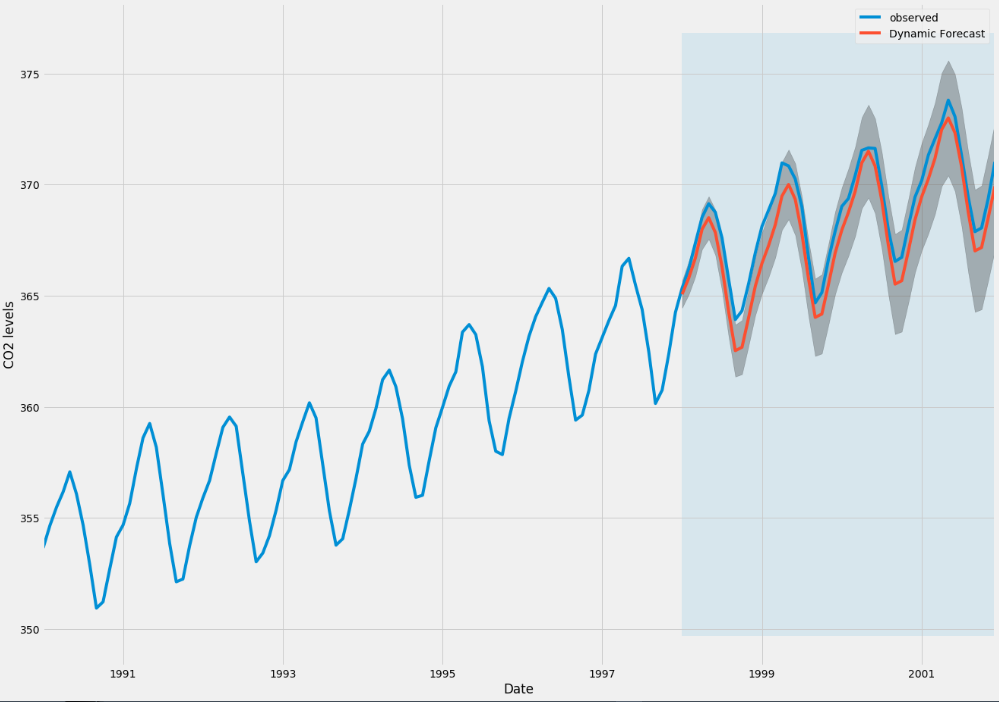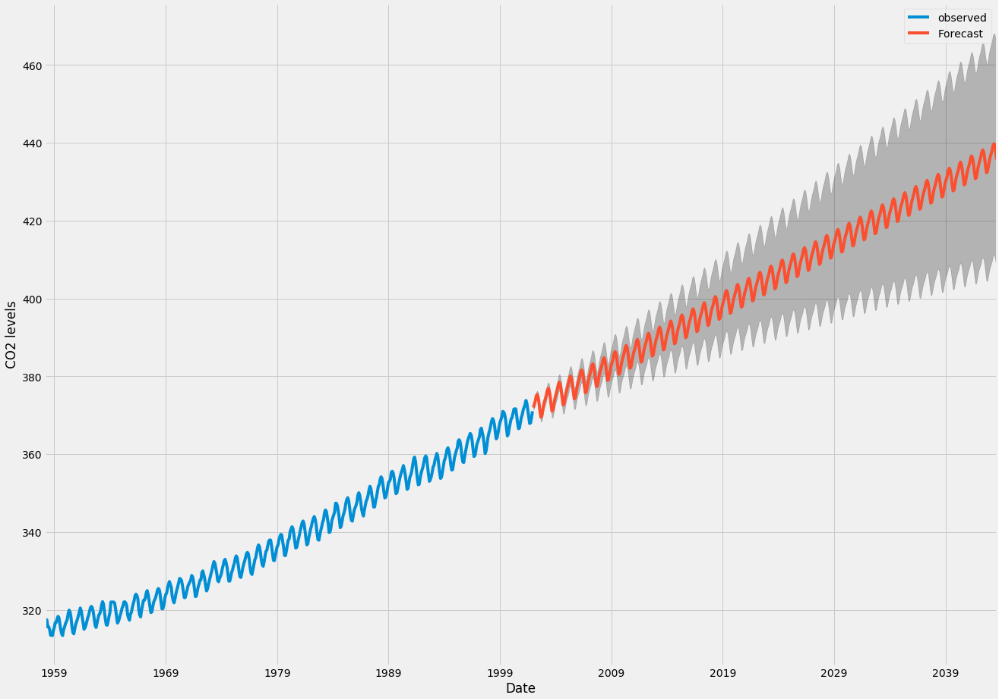# 结论

• 更改动态预测的开始日期，以了解这如何影响预测的整体质量。
• 尝试更多的参数组合，以查看是否可以提高模型的拟合优度。
• 选择其他指标选择最佳模型。例如，我们使用该 `AIC` 找到最佳模型。

Kaizong Ye拓端研究室（TRL）的研究员。

​非常感谢您阅读本文，如需帮助请联系我们！QQ在线咨询

15121130882

0571-63341498

## 关注有关新文章的微信公众号

This will close in 0 seconds